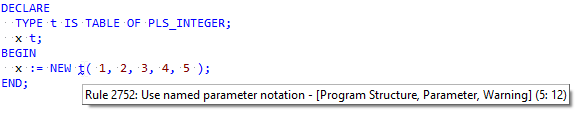# Rule 2752: "Use named parameter notation" for nested table constructor

I have no idea if you have the necessary information about the type, but this should not be reported:``````DECLARE
TYPE t IS TABLE OF PLS_INTEGER;
x t;
BEGIN
x := NEW t( 1, 2, 3, 4, 5 );
END;
``````

You're fully right.
I changed the 2752 expression to the following:

``````//PARAM_LIST [count(PARAM) >= (:MIN_PARAMS:)5(:/MIN_PARAMS:)]
[PARAM [not(PARAM_NAME)]]      (: at least one unnamed parameter :)
/parent::QNAME [not(parent::EXPR/@cat="type_constructor")]
/IDENTIFIER [last()]
[not(qp5:is-built-in-function(@value))]
``````

I also added a parameter MIN_PARAMS which will allow you to set the threshold.

Issue QP-4010. Expect it in the beta of the next few weeks.

Thanks for the feedback!
Andre

Thanks!
Looks like this would then fail to detect a type constructor (either record or object type) where parameters could/should be named though?

``````DECLARE
TYPE t IS RECORD ( x1 PLS_INTEGER, x2 PLS_INTEGER, x3 PLS_INTEGER, x4 PLS_INTEGER, x5 PLS_INTEGER );
v t;
BEGIN
v := NEW t( 1, 2, 3, 4, 5 );
END;
``````

Not sure what you mean.
A constructor with name parameters would look like this:

``````  v := NEW t( x1=>1, x2=>2, x3=>3, x4=>4, x5=>5 );
``````

or

``````  v := NEW t( 1, 2, x3=>3, x4=>4, x5=>5 );
``````

and none of those would be flagged, because it are constructors.

Or did I miss something?

I guess that your changed rule would not flag my latest example any more (because it is also a type constructor) .

It should be flagged though since named parameters are possible (as in your examples) but not used.

Right of course, in the RECORD case it looks like a method call, but the TABLE case fills up values. So my mod is not good yet.

Okay, will need a bit more work, let's see what can be done.

Hi Peter,
I came up with the following:

``````declare function TableOrVarrayTypeOfQName()
{
./(
let \$qname := .
return ./ancestor::BLOCK/STMT/DECL_SET/TYPE_SPEC[TYPE/@cat=("varray", "nested_table")]
[QNAME/@value=\$qname/IDENTIFIER/@value]
)
}

//PARAM_LIST [count(PARAM) >= (:MIN_PARAMS:)5(:/MIN_PARAMS:)]
[PARAM [not(PARAM_NAME)]]                (: at least one unnamed parameter :)
/parent::QNAME [not(TableOrVarrayTypeOfQName())]
/IDENTIFIER [last()]
[not(qp5:is-built-in-function(@value))]
``````

Here's some example text that I used (you can swap decl blocks by swapping the comments):

``````DECLARE
TYPE nt IS TABLE OF PLS_INTEGER;
my_nt nt;
TYPE va IS VARRAY (100) OF PLS_INTEGER;
my_va va;
TYPE rt IS RECORD ( x1 PLS_INTEGER, x2 PLS_INTEGER, x3 PLS_INTEGER, x4 PLS_INTEGER, x5 PLS_INTEGER );
my_rt rt;
BEGIN
BEGIN
DECLARE
PROCEDURE p
IS
--TYPE nt IS TABLE OF PLS_INTEGER;
--my_nt nt;
--TYPE va IS VARRAY (100) OF PLS_INTEGER;
--my_va va;
--TYPE rt IS RECORD ( x1 PLS_INTEGER, x2 PLS_INTEGER, x3 PLS_INTEGER, x4 PLS_INTEGER, x5 PLS_INTEGER );
--my_rt rt;
BEGIN
my_nt := nt( 1, 2, 3, 4, 5, 6 );
my_va := va( 1, 2, 3, 4, 5, 6 );
my_rt := rt( 1, 2, x3=>3, x4=>4, x5=>5 );
END;

BEGIN
NULL;
END;
END;
END;
``````

Only `rt` on the line `my_rt := rt( 1, 2, x3=>3, x4=>4, x5=>5 )` should be flagged.

Feel free to try it out. I'll keep the above as a fix, after some more testing.

Andre

Component QP5.dll version 5.391 now contains a new expression for this rule. Should be part of Toad soon.